﻿ Effect of Microwave Power and Sample Thickness on Microwave Drying Kinetics Elephant Foot Yam (Amorphophallus Paeoniifolius)

### Effect of Microwave Power and Sample Thickness on Microwave Drying Kinetics Elephant Foot Yam (Amorp...

Harish A, Vivek B S, Sushma R, Monisha J, Krishna Murthy T POPEN ACCESSPEER-REVIEWED

## Effect of Microwave Power and Sample Thickness on Microwave Drying Kinetics Elephant Foot Yam (Amorphophallus Paeoniifolius)

Harish A1, Vivek B S1, Sushma R1, Monisha J1, Krishna Murthy T P1,1Department of Biotechnology, Sapthagiri College of Engineering, Bangalore, India

### Abstract

Elephant foot yam was dried under different microwave power ranging from 180 W to 900 W and sample thickness (5-15 mm) to study their effect on microwave drying kinetics. Drying time, drying rate, kinetic rate constant, effective moisture diffusivity and rehydration ratio are various factors studied. Increase in microwave power and decrease in sample thickness increased drying rate and decrease the drying time. The Fick’s diffusion method was also used to model the experimental data. The effective moisture diffusivity values were found to be in the drying conditions range of 4.44 x 10-9 m2/s to 1.17 x 10-7 m2/s. The modified Arrhenius type equation was used to calculate the activation and the resulting range was from 23.47 to 9.23 Wg-1 for varying thickness of the sample. On the other hand, the interaction effect of drying conditions on the average drying rate, drying time, drying rate constant and effective moisture diffusivity with high significance has been explained by the second order quadratic polynomial model.

### At a glance: Figures

123
Prev Next

• A, Harish, et al. "Effect of Microwave Power and Sample Thickness on Microwave Drying Kinetics Elephant Foot Yam (Amorphophallus Paeoniifolius)." American Journal of Food Science and Technology 2.1 (2014): 28-35.
• A, H. , S, V. B. , R, S. , J, M. , & P, K. M. T. (2014). Effect of Microwave Power and Sample Thickness on Microwave Drying Kinetics Elephant Foot Yam (Amorphophallus Paeoniifolius). American Journal of Food Science and Technology, 2(1), 28-35.
• A, Harish, Vivek B S, Sushma R, Monisha J, and Krishna Murthy T P. "Effect of Microwave Power and Sample Thickness on Microwave Drying Kinetics Elephant Foot Yam (Amorphophallus Paeoniifolius)." American Journal of Food Science and Technology 2, no. 1 (2014): 28-35.

 Import into BibTeX Import into EndNote Import into RefMan Import into RefWorks

### 1. Introduction

Amorphophallus paeoniifolius is the scientific name of Elephant foot yam or White-spot giant arum. It belongs to the family Araceae  and has its origin from South East Asia. It is a tuber which is rich in starch, proteins and vitamins.

In Ayurvedic medicine  its roots are used to treat boils and opthalmia. Along with its uses as an thermogenic, analgesic, anthelmentic, liver tonic, diuretic and also for treating piles, dyspnoea, spleenomegaly and  it is also used as a staple food in several countries like Bangladesh, Indonesia, Sumatra, Malaysia, Philippines, Java, Srilanka, India, China and south eastern Asian countries [4, 5].

Drying is one of the oldest unit operation methods of preservation of agriculture materials having high moisture content. This complicated process involves simultaneous, coupled heat and mass transfer [6, 7]. It brings about substantial reduction in weight and volume thereby minimizing packaging, storage and transportation costs . Drying not only affects the moisture content of the product, but also alters other physical, biological and chemical properties of foods .

Many conventional thermal drying methods such as hot air drying, vacuum drying result in slow drying rates in the falling rate period of drying  and the long drying times at relatively high temperatures during the falling rate periods often lead to undesirable thermal degradation of the finished products and consume more energy and yield low drying efficiency . Microwave drying is having advantage of high drying rates, high energy efficiency, better product quality and efficient space utilization [12, 13, 14, 15].

Mathematical modeling of the drying process and equipment is an important aspect of drying technology in post-harvest processing of agricultural materials. Numerous mathematical equations can be found in literatures that describe drying phenomena of agricultural products. Among them, thin layer drying models have been found having wide application due to their ease of use and these models can be categorized as theoretical, semi-theoretical and empirical model [16, 17, 18].

The objective of present investigation is

•  To study the effect of microwave power and sample thickness on dying kinetics of elephant foot yam.

•  To compute effective moisture diffusivity and the activation energy at various drying conditions.

### 2. Materials and Methods

2.1. Experimental Material

Elephant foot yams were purchased from the local market at Yeshwanthpur, Bangalore, India. Roots were washed in running tap water to remove adhered soil and stored in refrigerator at 4 ± 1°C until taken for further processing. Three 50 g of sliced elephant foot yam were dried in hot air oven (Neha scientific international, Model no. SI 101A) at 105°C for 24hr to determine initial moisture content and the average initial moisture content of the fresh elephant foot yam was 4.204 kg H2O. kg db-1. The elephant foot yams were peeled and cut into rectangular slices having the dimensions of 50 x 20 x 5, 50 x 20 x 10, 50 x 20 x 15 mm using sharp stainless steel knives. Only rectangular specimens having thickness with in ±0.5 mm difference of the specific dimensions were selected for drying process.

2.2. Drying Procedure

Drying experiments were performed in domestic digital microwave oven (LG, India; Model MC-8087ABR) which has the capability of operating at five different microwave powers ranged from 180 to 900 Watts. Entire experiment was carried out using sliced elephant foot yam of known weight and arranged as thin layer on the rotatable plate fitted inside the microwave oven cabin. Drying was performed at a single power level and single thickness at a time. Weight loss was recorded at regular intervals of time using the digital weighing balance (CAS; Model MW-11-200 series) with an accuracy of 0.01 g. Drying experiments were conducted in triplicate and average values were reported.

2.3. Mathematical Modeling of Drying Kinetics

Experimental moisture content data of Elephant foot yam during microwave drying were converted to non-dimensionless moisture ratio using Eq. (1):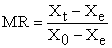(1)

The drying rate during the experiments was calculated using the following Eq. (2):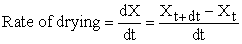(2)

Where Xo is the initial moisture content, Xt is the moisture content at time t and Xe is the equilibrium moisture content [9, 19]. Equation (1) can be further simplified to MR = Xt/ Xo as the values of Xe relatively small compared to Xo and Xt for long drying time, Xt+dt is the moisture content at time t+dt and Xt is the moisture content at time t and t is the drying time [20, 21, 22, 23].

The experimental data of dimensionless moisture ratio vs drying time were fitted to a semi empirical Midilli et.al model (Eq. 3) which is widely used to describe the drying behavior of agricultural materials to find the kinetic rate constant.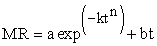(3)

Where k is kinetic rate constant (s-1) and a, b, n are model parameters respectively .

2.4. Calculation of Effective Moisture Diffusivity

Fick’s second law given in Eq. (4) was used to describe the drying process of the elephant foot yam.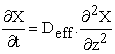(4)

Where X is the moisture content (kg. water. kg db-1), t is the time (s), z is the diffusion path (m), Deff is the moisture dependent diffusivity (m2/s) [16, 22, 25]. Analytical solution to Equation (3) was developed by  is given below [16, 18]:

The solution of the equation for infinite slab of thickness of 2 L is: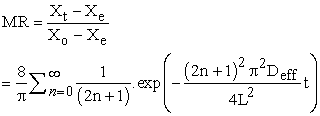(5)

For long drying period, Eq. (5) can be further simplified to only the first term of the series :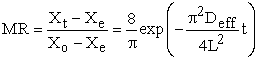(6)

Eq. (6) could be further simplified to a straight line equation as given below: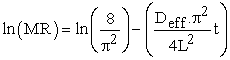(7)

Effective moisture diffusivity was typically determined by plotting experimental drying data in terms of ln (MR) Vs drying time and found from the slope (π2Deff/ 4 L2) according to Eq. (7).

2.5. Estimation of Activation Energy

For the calculation of activation energy, modified form of Arrhenius equation as derived by Dadali. et.al , illustrates the relationship between the kinetic rate constant and the ratio of the microwave power output to sample weight instead of temperature and the equation (Eq. 8) is described below: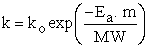(8)

Where ko (s-1) is the pre-exponential factor, Ea is the activation energy (W.g-1), MW is the microwave power output (W), m is the mass of the sample (g).

2.6. Rehydration Studies

Dried elephant foot yam slices were rehydrated by immersing in distilled water at room temperature. About 5 g of dried samples were placed in glass beakers containing water in ratio 1:25 (w/w) for 8 hr. Samples were drained, blotted with tissue paper and weighed. The rehydration capacity was calculated as follows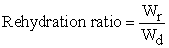(9)

Where Wr and Wd are weight after the rehydration and weight before rehydration respectively .

2.7. Statistical Analysis

Nonlinear Least square method using the SOLVER tool based on the Generalized Reduced Gradient (GRG) method of iteration available in Microsoft Excel (Microsoft Office 2010, USA) was used to fit the experimental data to selected model. Goodness of fit was evaluated by coefficient of determination (R2) (Eq. 10) as primary criterion. Statistical parameters may be computed from the following mathematical equations.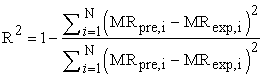(10)

Where N is the total number of observations, p is number of factors in the mathematical model, MRexp,i and MRpre,i are (the experimental and predicted moisture ratio at any observation i) [15, 29] coefficients for the second order quadratic polynomial model Eq. (11) to study the interaction effect of drying parameters and ANOVA was done in Microsoft Excel data analysis. Three-dimensional response contour plots were obtained using Kyplot software (software developed by Koichi Yoshioka and available online:

http://www.woundedmoon.org/win32/kyplot. html).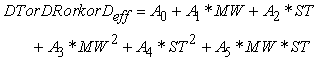(11)

Where DT is drying time, DR is average drying rate, k is kinetic rate constant, Deff is effective moisture diffusivity, MW is microwave power and ST is sample thickness. A0 to A5 are the coefficients of the model.

### 3. Results and Discussions

3.1. Effect of Microwave Power and Thickness on Drying Kinetics
Figure 1. Moisture ratio vs drying time at various microwave powers (a) 5 mm (b) 10 mm (c) 15 mm

Known weight elephant foot yam was dried at five microwave power output (900 to 180 W) and three different sample thicknesses (5, 10, 15 mm) in microwave dryer to study the effect of microwave power and sample thickness on moisture content, drying rate and drying time, effective moisture diffusivity and rehydration ratio. Drying curves of moisture ratio vs. drying time reflecting the effect of microwave power and sample thicknesses are shown in Figure 1 (a-c). Drying time to reduce the initial moisture content of 4.204 kg H2O.kg db1 to 0.01 kg H2O. kg db1 decreases significantly as the microwave power increases from 180 W to 900 W and decreases in sample thickness from 15 mm to 5 mm. Drying time is directly proportional to sample thickness and inversely proportional to the microwave power.

Figure 2 (a-c) show the drying rate calculated using Eq. (2) against the time at different drying conditions. Initially there is sudden increase in the drying rate and there is no constant drying rate period in the present investigation but a short accelerating period at the start. Initially dehydration rate is very high, as seen from the Figure 3 (a-c) to remove 50% of the moisture, it takes 25-30% of the total drying time. Drying rate decreased rapidly with decrease in the moisture content and took more time to remove final moisture content. The average drying rates were in the range of 1.2 x 10-3 to 9.08 x 10-3 kg H2O.kg db1 at selected experimental conditions.

#### Table 2. ANOVA and regression coefficients of the fitted equation and standard errors for drying Rate

To study the interaction effect of microwave power and sample thickness on drying time and average drying rate, the experimental data was fitted to a second order quadratic polynomial equation. Multiple regression analysis was performed to obtain the model constants. The model was built based on the variables with 5% confidence level. The ANOVA results and coefficient values were reported in Table 1 and Table 2 for drying time and average drying rate respectively and indicate that the model is highly significant. Furthermore a higher value of R2 and Adjusted R2 indicates a high dependency and correlation between the observed and the predicted values of drying rate and drying time. As seen from Table 1 the effect of linear factors microwave power and sample thickness was found to be highly significant (P = 0.0002 and p = 0.00078 for microwave power and sample thickness respectively) on the drying time. The square terms of the microwave power is significant (p = 0.0023) where as the square term of sample thickness is not significant (P > 0.05). The interaction terms of microwave power and sample thickness is also found to be significant (p = 0.002). From Table 2 the effect of linear terms and the square terms of microwave power and sample thickness were not found to be significant (p > 0.05) but the interaction of microwave power and sample thickness found to be highly significant (p = 0.0026) on average drying rate. The negative sign of the coefficient means antagonistic effect, increase in that parameter decreases the value of response. The positive sign of coefficient represents the synergistic effect, increase in that parameter increases the value of response . Figure 4 and Figure 5 describe the interactive effect of microwave power and sample thickness on drying time and average dying rate respectively.

3.3. Effect of Microwave Power and Thickness on Kinetic Rate Constant

The drying rate constant obtained from Midilli et al. model was in the range of 0.0015 to 0.19 min-1. Kinetic or drying rate constant was found to be increased with increase in microwave power and decreased with increase in the Elephant foot yam sample thickness. This implies that increase in the microwave power and decrease in sample thickness increases the moisture removal rate. To study the interaction effect of microwave power and sample thickness on drying rate constant, the data was fitted to the second order quadratic polynomial equation. The model was built based on the variables with confidence levels of 5%. The analysis of variance (ANOVA) reported in Table 3 indicates that the model is highly significant, which is evident from the high Fisher (F) ratio value and low probability value. The effect of linear factor microwave power (P = 0.0009) on the drying rate constant is found to be significant. The linear term of sample thickness is not significant (P > 0.05) on drying rate constant. The square terms of the microwave power is significant (P = 0.0045) and sample thickness was not found to be significant (P > 0.05) on drying rate constant. The interaction terms of microwave power and sample thickness were found to highly significant (p = 1.97 x 10-6) when compared to the individual factor effect on the drying rate constant. The constant corresponding to the microwave power is positive and it indicates a positive effect on drying rate constant and as well an increase in microwave power output, which in turn increases the rate of drying. And negative value of sample thickness indicates the negative effect in which increase in sample thickness decreases the drying rate during the microwave drying of elephant foot yam. Furthermore, high values of R2 (0.9943) and R2 (adjusted) (0.9823) indicates a high dependence and correlation between the observed and the predicted values of the drying rate constant. Figure 6 describes the interactive effect of microwave power and sample thickness on dying rate constant.

#### Table 4. Effective moisture diffusivities of elephant foot yam at different microwave power and sample thickness

3.4. Effect of Microwave Power and Thickness on Effective Moisture Diffusivity

The effective moisture diffusivities of elephant foot yam undergoing microwave drying were calculated according to Eq. (7) and are presented in Table 4. Effective moisture diffusivity values of elephant foot yam under various drying conditions were estimated in the range of 4.44 x 10-9 m2/s to 1.17 x 10-7 m2/s. The values were within the range of 10-6 to 10-12 m2/s for drying of food materials [30, 31]). The Effective moisture diffusivity increased with increase in microwave power and sample thickness. To study the interaction effect of microwave power and sample thickness on Effective moisture diffusivity, the data was fitted to the second order quadratic polynomial equation. The model was built based on the variables with confidence levels of 5%. ANOVA result shows the model is significant. From Table 5 it found that, the Linear and quadratics terms of microwave power and sample thickness were not found to be significant (P > 0.05). Whereas the interaction between the microwave power and sample thickness on the effective moisture diffusivity is significant (P = 0.0010). Furthermore, high values of R2 (0.975) and R2 (adjusted) (0.966) indicates a high dependence and correlation between the observed and the predicted values of the drying rate constant.

#### Table 6. Calculated Activation energy at different sample thickness

3.5. Effect of Thickness on Activation Energy

The kinetic rate constant (k) values regress well with ratio of sample mass to microwave power (m/MW) value based on Eq. (8). The coefficient of determination (R2), activation energy and pre-exponential factor values are presented in Table 6. In the present study the activation energy during microwave drying of Elephant foot yam decreased with increase in sample thickness. A correlation has developed between activation energy and sample thickness, the exponential model fits best to the data with R2 value of 0.9856 and the regression equation is given in Eq. (12):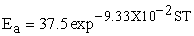(12)

Where Ea (W.g-1) is the activation energy and ST (mm) is the sample thickness.

3.6. Effect of Microwave Power and Sample Thickness on Rehydration Ratio

Values of Rehydration ratio (RR) for elephant foot yam samples dried in various conditions were found to be in the range of 1.76–2.67. The ANOVA results presented in the Table 7 shows that the sample thickness significantly affect the Rehydration ratio (P = 2.28 x 10-5) where as the microwave power does not have any significant effect on the rehydration ratio. The Figure 7 explains the dependency of rehydration ratio on microwave power and sample thickness.

#### Table 7. Analysis of Variance (ANOVA) for Rehydration ratio

Figure 7. Effect of microwave power and sample thickness on rehydration ratio

### 4. Conclusion

The drying time, drying rate, drying rate constant, effective moisture diffusivity and rehydration ratio of elephant foot yam were investigated as functions of microwave power and sample thickness. This helps in the optimization and simulation of drying process. The kinetic rate constant obtained from the Midilli et al. model increased with increase in microwave power and decreased with increase in sample thickness. The values of effective moisture diffusivity, calculated using Fick’s diffusion for drying experiments at 180-900 W and 5-15 mm sample thickness ranged from 4.44 x 10-9 m2/s to 1.17 x 10-7 m2/s. The effective moisture diffusivity increased with increase in microwave power and sample thickness. The second order quadratic polynomial model was built to study the interaction effect of microwave power on drying time, average drying rate, drying rate constant, effective moisture diffusivity. All the model were significant and explains the high dependency and correlation between the

observed and predicted values of drying time, average drying rate, drying rate constant, effective moisture diffusivity. Rehydration Ratio increased with decrease in sample thickness and Microwave power has no significant effect on it.

### References

  Hedrick, U.P., 1972. Sturtevant’s Edible Plants of the World. Dover Publications; Mineola, NY, USA 686.In article  Angayarkanni, J., Ramkumar, K.M., Poornima, T., Priyadarshini, U., 2007. Cytotoxicity Activity of Amorphophallus paeoniifolius tuber extracts In vitro. Journal of Agricultural and Environmental Science., 2 (4): 395-398.In article  Hurkadle, P.J., Palled, S.G., Shelar, P.A., Mandavkar, Y.D., Khedkar, A.S., 2012. Hepatoprotective activity of Amorphophallus paeoniifolius tubers against paracetamol-induced liver damage in rats. Journal of Tropical Biomedicine., S238-S242.In article  Chandra, S., 1984. Edible aroids Clarendor Press Oxford.In article  Ravi, V., Ravindran, C.S., Suja, G., 2009. Growth and Productivity of Elephant Foot Yam (Amorphophallus paeoniifolius) (Dennst.Nicolson): an Overview. Journal of Root Crops., 35: 131-142.In article  Diamante, L.M., Ihns, R., Savage, G.P., Vanhanen, L., 2010. A new mathematical model for thin layer drying of fruits. International Journal of Food Science and Technology., 45 (9): 1956-1962.In article CrossRef  Karimi, F., Rafiee, S., Taheri-garavanda, A., Karimi, M., 2012. Optimization of an air drying process for Artemisia absinthiun leaves using response surface and artificial neural network models. Journal of Taiwan International Chemical Engineering., 43 (1): 29-39.In article CrossRef  Okos, M.R., Narsimhan, G., Singh, R.K., Witnauer, A.C., 1992. Food dehydration: In Heldman, D.R., Lund, D.B., edn. Handbook of food engineering Marcel Dekker, New York.In article  Ozbek, B., Dadali, G., 2007. Thin-layer drying characteristics and modeling of mint leaves undergoing microwave treatment. Journal of Food Engineering., 83 (4): 541-549.In article CrossRef  Zhang, M., Tang, J., Mujumdar, A.S., Wang, S., 2006. Trends in microwave related drying of fruits and vegetables. Trends Food Science and Technology., 17: 524-534.In article CrossRef  Alibas ozkan, I., Akbudak, B., Akbudak, N., 2007. Microwave drying characteristics of spinach. Journal of Food Engineering., 78 (2): 577-583.In article  Sutar, P.P., Prasad, S., 2007. Modeling microwave vacuum drying kinetics and moisture diffusivity of carrot slices. Drying Technology., 25 (10): 1695-1702.In article CrossRef  Kumar, D., Prasad, S., Murthy, S.G., 2011. Optimization of microwave-assisted hot air drying conditions of okra using response surface methodology. Journal of Food Science and Technology.In article  Dadali, G., Apar, D.K., Ozbek, B., 2007. Estimation of effective moisture diffusivity of okra for microwave drying. Drying Technology., 25 (5): 1445-1450.In article CrossRef  Krishna Murthy, T.P., Manohar, B., 2013. Hot air drying characteristics of mango ginger: Prediction of drying kinetics by Mathematical Modeling and artificial neural network. Journal of Food Science and Technology.In article  Akpinar, E.K., 2006. Determination of suitable thin layer drying curve model for some vegetables and fruits. Journal of Food Engineering., 73 (1): 75-84.In article CrossRef  Midilli, A., Kucuk, H., Yapar, Z.A., 2002. New model for single layer drying. Drying Technology., 20 (7): 1503-1513.In article CrossRef  Park, K.J., Vohnikova, Z., Bord, F.P.R., 2002. Evaluation of drying parameters and desorption isotherms of garden mint leaves. Journal of Food Engineering., 51 (3): 193-199.In article CrossRef  Ertekin, C., Yaldiza, O., 2004. Drying of eggplant and selection of a suitable thin layer drying model. Journal of Food Engineering., 63 (3): 349-359.In article CrossRef  Diamante, L.M., Munro, P.A., 1993. Mathematical modeling of hot air drying of sweet potato slices. International Journal of Food Science and Technology., 26 (1): 99-109.In article CrossRef  Esturk, O., 2010. Intermittent and Continuous microwave-convective air drying characteristics of sage (Salvia officinalis) leaves. Journal of Food Bioprocessing and Technology., 5 (5): 1664-1673.In article CrossRef  Wang, J., Wang, J. S., Yu, Y., 2007. Microwave drying characteristics and dried quality of pumpkin. International Journal of Food Science and Technolongy., 42 (2): 148-156.In article CrossRef  Doymanz, I., Akgun, N.A., 2009. Study of Thin-Layer Drying of Grape Wastes. Journal of Chemical Engineering, 196 (7), 890-900.In article  Midilli, A., Kucuk, H., (2003). Mathematical Modeling of thin layer drying of pistachio by using solar energy. Energy Conversion and Management., 44 (7): 1111-1122.In article CrossRef  Doymaz, I., 2004. Convective air drying characteristics of thin layer carrots. Journal of Food Engineering., 61 (3): 359-364.In article CrossRef  Crank, J., 1975. The Mathematics of Diffusion, 2nd edn. Oxford University press, London. 267-268.In article  Tutuncu, M.A., Labuza, T.P., 1996. Effect of geometry on the effective moisture transfer diffusion coefficient. Journal of Food Engineering., 30 (3-4): 433-447.In article CrossRef  Doymaz, I., Ismail, O., 2012. Modeling of rehydration kinetics of green bell peppers. Journal of food processing and Preservation., 37 (5): 907-913.In article  Krishna Murhty, T.P., Manohar, B., 2012. Microwave drying of mango ginger (Curcuma amada roxb): prediction of drying kinetics by mathematical modeling and artificial neural network. International Journal of Food Science and Technology., 47 (6): 1229-1236.In article CrossRef  Zogzas, N.P., Maroulis, Z.B., Marinos-kouris, D., 1996. Moisture Diffusivity Data Compilation in Foodstuffs. Drying Technology 14 (10), 2225-2253.In article CrossRef  Falade, K.O., Solademi, O. J., 2010. Modelling of Air drying of fresh and blanched sweet potato slices. International Journal of Food Science and Technology 45 (2), 278-288.In article CrossRef